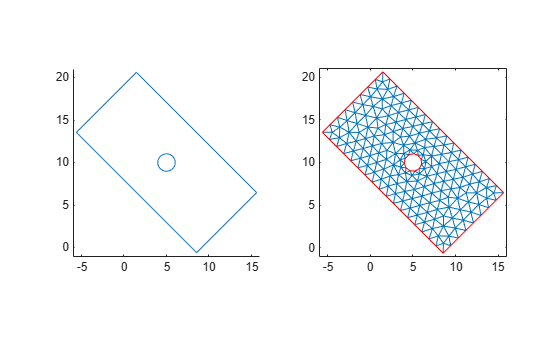# rotate

Rotate geometry

## Syntax

``rotate(g,theta)``
``rotate(g,theta,refpoint)``
``rotate(g,theta,refpoint1,refpoint2)``
``h = rotate(g,___)``

## Description

example

````rotate(g,theta)` rotates the geometry `g` about the z-axis by the angle `theta`, specified in degrees. Rotation follows the right-hand rule: a positive angle `theta` rotates counterclockwise, while sighting along the z-axis toward the origin.```
````rotate(g,theta,refpoint)` uses the rotation axis specified by the reference point `refpoint`. The axis of rotation is the line in the z-direction passing through the reference point.```

example

````rotate(g,theta,refpoint1,refpoint2)` uses the rotation axis specified by two reference points. This syntax is only valid for a 3-D geometry.```
````h = rotate(g,___)` returns a handle `h` to the resulting geometry object `g`. Use this syntax with any input arguments from the previous syntaxes. If the original geometry is a `DiscreteGeometry` object, then the function modifies the original geometry and returns the handle `h` to the modified `DiscreteGeometry` geometry object. If the original geometry is an `AnalyticGeometry` object, then `h` is a handle to a new `DiscreteGeometry` geometry object. In this case, the original geometry remains unchanged.```

## Examples

collapse all

Rotate a geometry with and without specifying the reference point for the axis of rotation.

Create a model.

`model = createpde;`

Import and plot a geometry.

```g = importGeometry(model,'PlateHolePlanar.stl'); pdegplot(g)```Mesh the geometry and plot the mesh.

```generateMesh(model); figure pdemesh(model)```Rotate the geometry around the default z-axis by 45 degrees. Plot the result.

```rotate(g,45); figure pdegplot(g)```Plot the geometry and mesh. The `rotate` function modifies a geometry, but it does not modify a mesh.

```figure pdegplot(g) hold on pdemesh(model)```After modifying the geometry, always regenerate the mesh.

```generateMesh(model); figure pdegplot(g) hold on pdemesh(model)```Restore the original geometry position.

`rotate(g,-45);`

Rotate the geometry by the same angle, but this time use the center of the geometry as a reference point. The axis of rotation is the line in the z-direction passing through the reference point.

`rotate(g,45,[5 10]);`

Regenerate the mesh.

`generateMesh(model);`

Plot the resulting geometry and mesh.

```figure subplot(1,2,1) pdegplot(model) axis([-6 16 -1 21]) subplot(1,2,2) pdemesh(model) axis([-6 16 -1 21])```Rotate a geometry with and without specifying the reference points for the axis of rotation.

Create and plot a geometry.

```g = multicuboid(1,5,1); pdegplot(g)```Rotate a 3-D geometry around the default z-axis by 45 degrees. Plot the result.

```rotate(g,45); pdegplot(g)```Restore the original geometry position.

```rotate(g,-45); pdegplot(g)```Rotate the geometry by the same angle, but this time around the y-axis.

```rotate(g,45,[0 0 0],[0 1 0]); pdegplot(g)```## Input Arguments

collapse all

Geometry, specified as a `DiscreteGeometry` or `AnalyticGeometry` object.

Example: `g = model.Geometry`

Rotation angle in degrees, specified as a real number.

Example: `rotate(g,90)`

Reference point for a rotation axis, specified as a vector of two or three real numbers. The axis of rotation is the line in the z-direction passing through the reference point.

Example: `rotate(g,45,[1 1.5])`

Reference points that define a rotation axis for a 3-D geometry, specified as a vector of three real numbers.

Example: `rotate(g,45,[0 0 0],[1 1 1])`

## Output Arguments

collapse all

Resulting geometry, returned as a handle. If the original geometry `g` is a `DiscreteGeometry` object, then `h` is a handle to the modified `DiscreteGeometry` geometry object `g`. If `g` is an `AnalyticGeometry` object, then `h` is a handle to a new `DiscreteGeometry` geometry object. In this case, the original geometry `g` remains unchanged.

## Tips

• `rotate` modifies a geometry, but it does not modify a mesh. After modifying a geometry, regenerate the mesh to ensure a proper mesh association with the new geometry.

• If `g` is an `AnalyticGeometry` object, and you want to replace it with the resulting discrete geometry, assign the output to the original geometry, for example, `g = rotate(g,90)`.